# Chapter 6: Structures

%load_ext autoreload
%matplotlib inline
%config InlineBackend.figure_format = 'retina'
import warnings
warnings.filterwarnings('ignore')


## Introduction

from IPython.display import YouTubeVideo



If you remember, at the beginning of this book, we saw a quote from John Quackenbush that essentially said that the reason a graph is interesting is because of its edges. In this chapter, we'll see this in action once again, as we are going to figure out how to leverage the edges to find special structures in a graph.

## Triangles

The first structure that we are going to learn about is triangles. Triangles are super interesting! They are what one might consider to be "the simplest complex structure" in a graph. Triangles can also have semantically-rich meaning depending on the application. To borrow a bad example, love triangles in social networks are generally frowned upon, while on the other hand, when we connect two people that we know together, we instead complete a triangle.

To learn about triangles, we are going to leverage a physician trust network. Here's the data description:

This directed network captures innovation spread among 246 physicians for towns in Illinois, Peoria, Bloomington, Quincy and Galesburg. The data was collected in 1966. A node represents a physician and an edge between two physicians shows that the left physician told that the right physician is his friend or that he turns to the right physician if he needs advice or is interested in a discussion. There always only exists one edge between two nodes even if more than one of the listed conditions are true.

from nams import load_data as cf


### Exercise: Finding triangles in a graph

This exercise is going to flex your ability to "think on a graph", just as you did in the previous chapters.

Leveraging what you know, can you think of a few strategies to find triangles in a graph?

from nams.solutions.structures import triangle_finding_strategies

# triangle_finding_strategies()


### Exercise: Identify whether a node is in a triangle relationship or not

Let's now get down to implementing this next piece of code.

Write a function that identifies whether a node is or is not in a triangle relationship. It should take in a graph G and a node n, and return a boolean True if the node n is in any triangle relationship and boolean False if the node n is not in any triangle relationship.

Every graph object G has a G.has_edge(n1, n2) method that you can use to identify whether a graph has an edge between n1 and n2.

Also:

itertools.combinations lets you iterate over every K-combination of items in an iterable.

def in_triangle(G, node):
pass

from nams.solutions.structures import in_triangle

# UNCOMMENT THE NEXT LINE TO SEE MY ANSWER
# in_triangle??


Now, test your implementation below! The code cell will not error out if your answer is correct.

from random import sample
import networkx as nx

def test_in_triangle():
nodes = sample(G.nodes(), 10)
for node in nodes:
assert in_triangle(G, 3) == bool(nx.triangles(G, 3))

test_in_triangle()


As you can see from the test function above, NetworkX provides an nx.triangles(G, node) function. It returns the number of triangles that a node is involved in. We convert it to boolean as a hack to check whether or not a node is involved in a triangle relationship because 0 is equivalent to boolean False, while any non-zero number is equivalent to boolean True.

### Exercise: Extract triangles for plotting

We're going to leverage another piece of knowledge that you already have: the ability to extract subgraphs. We'll be plotting all of the triangles that a node is involved in.

Given a node, write a function that extracts out all of the neighbors that it is in a triangle relationship with. Then, in a new function, implement code that plots only the subgraph that contains those nodes.

def get_triangle_neighbors(G, n):
pass

from nams.solutions.structures import get_triangle_neighbors

# UNCOMMENT THE NEXT LINE TO SEE MY ANSWER
# get_triangle_neighbors??

def plot_triangle_relations(G, n):
pass

from nams.solutions.structures import plot_triangle_relations

plot_triangle_relations(G, 3)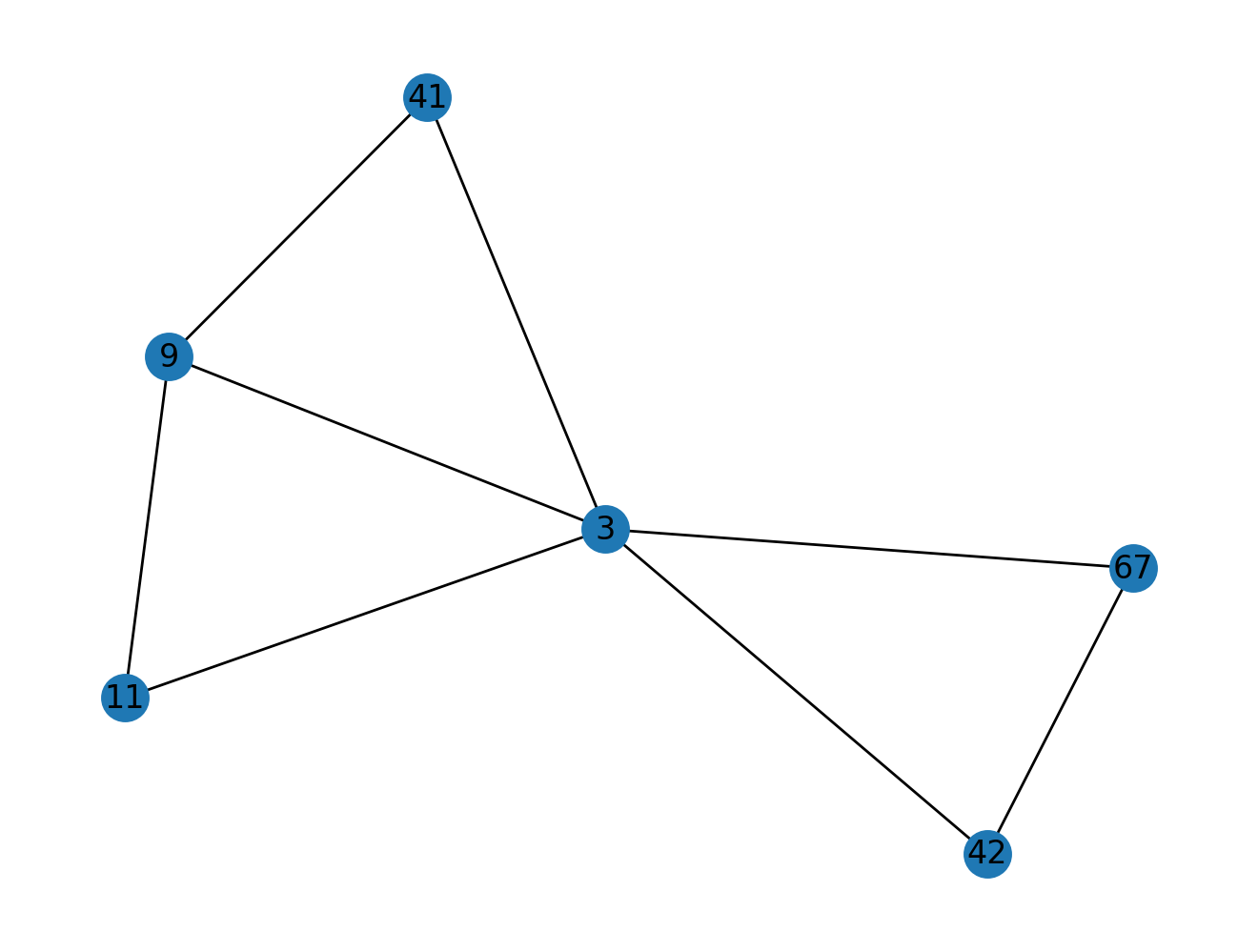In professional circles, making connections between two people is one of the most valuable things you can do professionally. What you do in that moment is what we would call triadic closure. Algorithmically, we can do the same thing if we maintain a graph of connections!

Essentially, what we are looking for are "open" or "unfinished" triangles".

In this section, we'll try our hand at implementing a rudimentary triadic closure system.

### Exercise: Design the algorithm

What graph logic would you use to identify triadic closure opportunities? Try writing out your general strategy, or discuss it with someone.

from nams.solutions.structures import triadic_closure_algorithm



Write a function that takes in a graph G and a node n, and returns all of the neighbors that are potential triadic closures with n being the center node.

def get_open_triangles_neighbors(G, n):
pass

from nams.solutions.structures import get_open_triangles_neighbors

# UNCOMMENT THE NEXT LINE TO SEE MY ANSWER
# get_open_triangles_neighbors??


### Exercise: Plot the open triangles

Now, write a function that takes in a graph G and a node n, and plots out that node n and all of the neighbors that it could help close triangles with.

def plot_open_triangle_relations(G, n):
pass

from nams.solutions.structures import plot_open_triangle_relations

plot_open_triangle_relations(G, 3)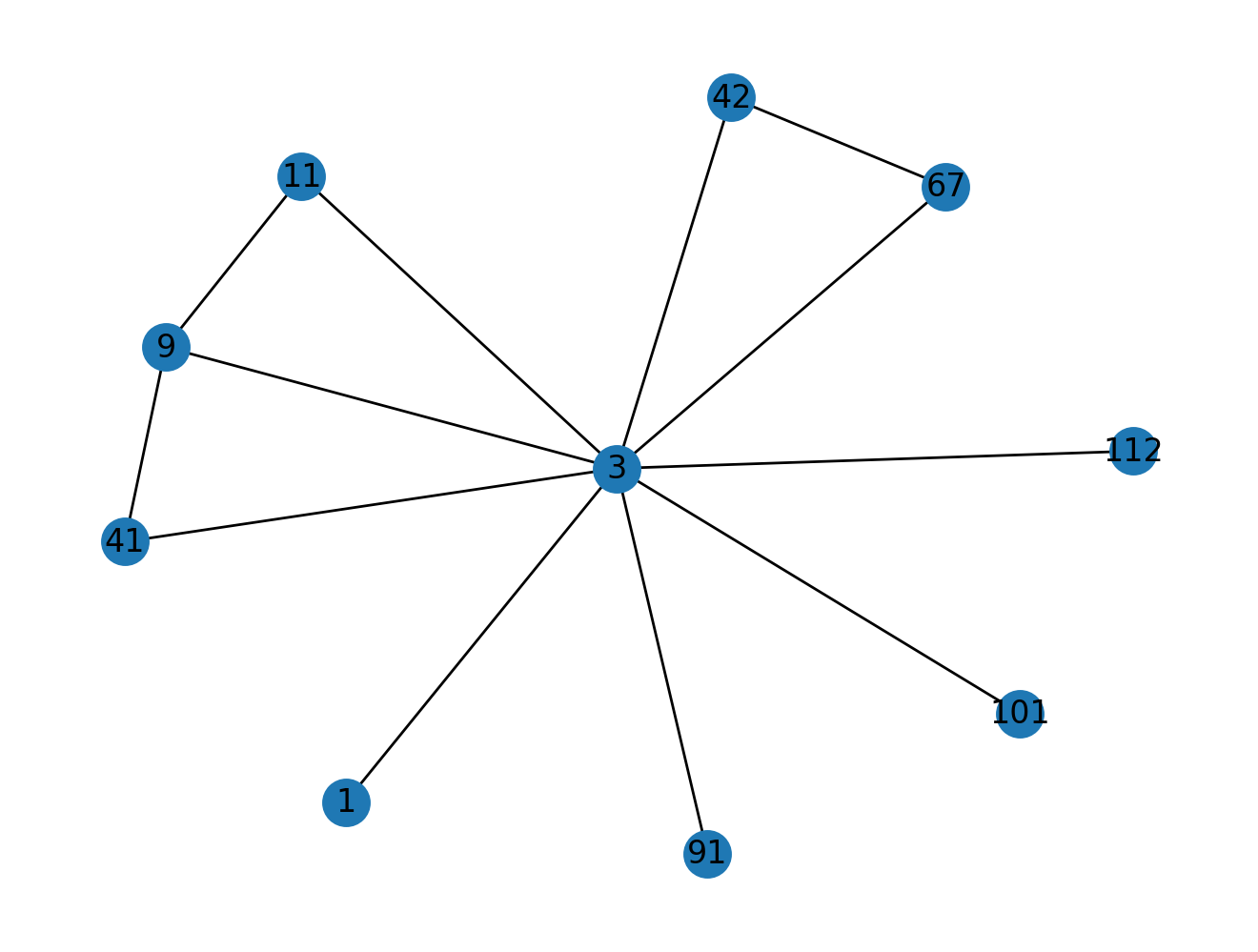## Cliques

Triangles are interesting in a graph theoretic setting because triangles are the simplest complex clique that exist.

But wait! What is the definition of a "clique"?

A "clique" is a set of nodes in a graph that are fully connected with one another by edges between them.

### Exercise: Simplest cliques

Given this definition, what is the simplest "clique" possible?

from nams.solutions.structures import simplest_clique

# UNCOMMENT THE NEXT LINE TO SEE MY ANSWER
# simplest_clique()


### $k$-Cliques

Cliques are identified by their size $k$, which is the number of nodes that are present in the clique.

A triangle is what we would consider to be a $k$-clique where $k=3$.

A square with cross-diagonal connections is what we would consider to be a $k$-clique where $k=4$.

By now, you should get the gist of the idea.

### Maximal Cliques

Related to this idea of a $k$-clique is another idea called "maximal cliques".

Maximal cliques are defined as follows:

A maximal clique is a subgraph of nodes in a graph

1. to which no other node can be added to it and
2. still remain a clique.

NetworkX provides a way to find all maximal cliques:

# I have truncated the output to the first 5 maximal cliques.
list(nx.find_cliques(G))[0:5]

[[1, 2], [1, 3], [1, 4, 5, 6], [1, 7], [1, 72]]


### Exercise: finding sized-$k$ maximal cliques

Write a generator function that yields all maximal cliques of size $k$.

I'm requesting a generator as a matter of good practice; you never know when the list you return might explode in memory consumption, so generators are a cheap and easy way to reduce memory usage.

def size_k_maximal_cliques(G, k):
pass

from nams.solutions.structures import size_k_maximal_cliques


Now, test your implementation against the test function below.

def test_size_k_maximal_cliques(G, k):
clique_generator = size_k_maximal_cliques(G, k)
for clique in clique_generator:
assert len(clique) == k

test_size_k_maximal_cliques(G, 5)


### Clique Decomposition

One super neat property of cliques is that every clique of size $k$ can be decomposed to the set of cliques of size $k-1$.

Does this make sense to you? If not, think about triangles (3-cliques). They can be decomposed to three edges (2-cliques).

Think again about 4-cliques. Housed within 4-cliques are four 3-cliques. Draw it out if you're still not convinced!

### Exercise: finding all $k$-cliques in a graph

Knowing this property of $k$-cliques, write a generator function that yields all $k$-cliques in a graph, leveraging the nx.find_cliques(G) function.

If a $k$-clique can be decomposed to its $k-1$ cliques, it follows that the $k-1$ cliques can be decomposed into $k-2$ cliques, and so on until you hit 2-cliques. This implies that all cliques of size $k$ house cliques of size $n < k$, where $n >= 2$.

def find_k_cliques(G, k):
pass

from nams.solutions.structures import find_k_cliques

def test_find_k_cliques(G, k):
for clique in find_k_cliques(G, k):
assert len(clique) == k

test_find_k_cliques(G, 3)


## Connected Components

Now that we've explored a lot around cliques, we're now going to explore this idea of "connected components". To do so, I am going to have you draw the graph that we are working with.

import nxviz as nv

nv.circos(G)

<AxesSubplot:>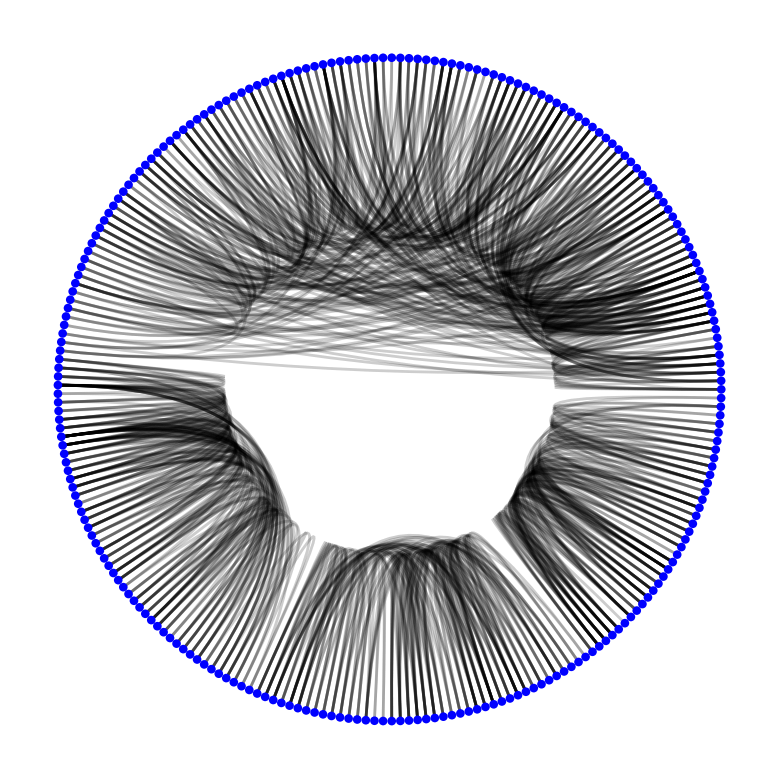### Exercise: Visual insights

From this rendering of the CircosPlot, what visual insights do you have about the structure of the graph?

from nams.solutions.structures import visual_insights

# UNCOMMENT TO SEE MY ANSWER
# visual_insights()


### Defining connected components

From Wikipedia:

In graph theory, a connected component (or just component) of an undirected graph is a subgraph in which any two vertices are connected to each other by paths, and which is connected to no additional vertices in the supergraph.

NetworkX provides a function to let us find all of the connected components:

ccsubgraph_nodes = list(nx.connected_components(G))


Let's see how many connected component subgraphs are present:

len(ccsubgraph_nodes)

4


### Exercise: visualizing connected component subgraphs

In this exercise, we're going to draw a circos plot of the graph, but colour and order the nodes by their connected component subgraph.

Recall Circos API:

c = CircosPlot(G, node_order='node_attribute', node_color='node_attribute')
c.draw()
plt.show()  # or plt.savefig(...)


Follow the steps along here to accomplish this.

Firstly, label the nodes with a unique identifier for connected component subgraph that it resides in. Use subgraph to store this piece of metadata.

def label_connected_component_subgraphs(G):
return G

from nams.solutions.structures import label_connected_component_subgraphs
G_labelled = label_connected_component_subgraphs(G)

# UNCOMMENT TO SEE THE ANSWER
# label_connected_component_subgraphs??


Now, draw a CircosPlot with the node order and colouring dictated by the subgraph key.

def plot_cc_subgraph(G):
pass

from nams.solutions.structures import plot_cc_subgraph
from nxviz import annotate

plot_cc_subgraph(G_labelled)
annotate.circos_group(G_labelled, group_by="subgraph")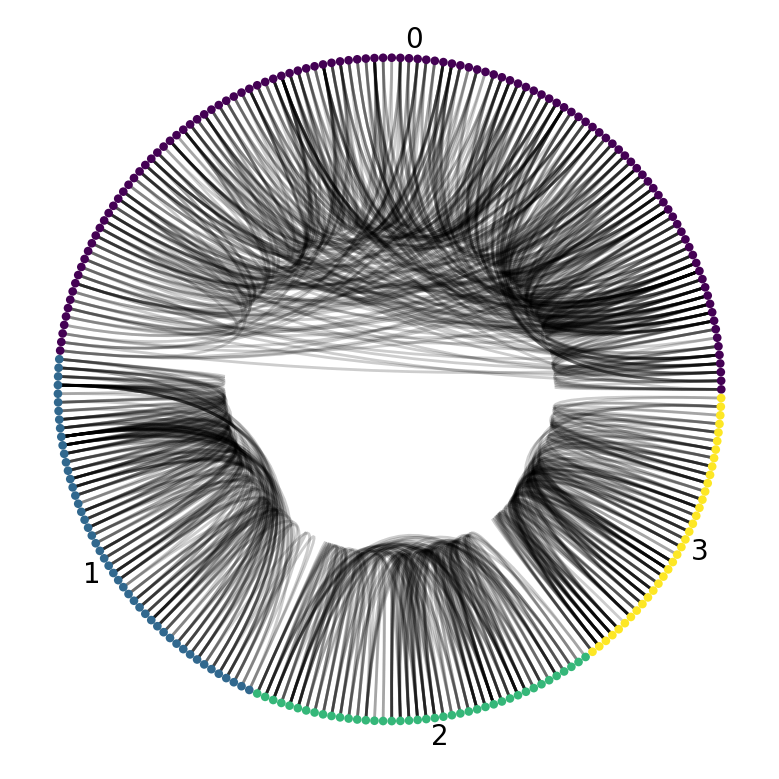Using an arc plot will also clearly illuminate for us that there are no inter-group connections.

nv.arc(G_labelled, group_by="subgraph", node_color_by="subgraph")
annotate.arc_group(G_labelled, group_by="subgraph", rotation=0)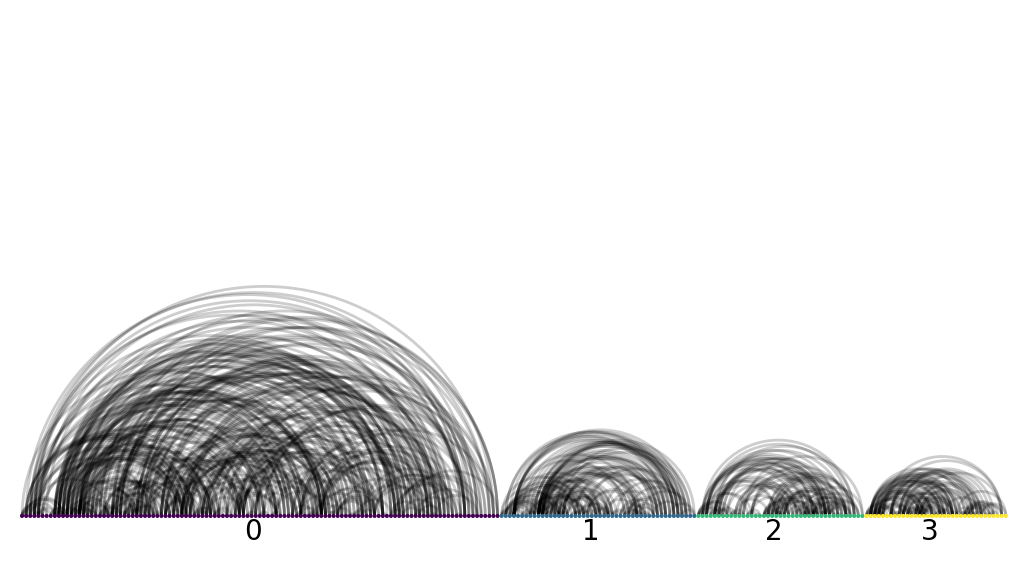Voila! It looks quite clear that there are indeed four disjoint group of physicians.

## Solutions

from nams.solutions import structures
import inspect

print(inspect.getsource(structures))

"""Solutions to Structures chapter."""

from itertools import combinations

import networkx as nx
from nxviz import circos
from nams.functions import render_html

def triangle_finding_strategies():
"""
How to find triangles.
"""
ans = """
One way would be to take one node, and look at its neighbors.
If its neighbors are also connected to one another,
then we have found a triangle.

Another way would be to start at a given node,
and walk out two nodes.
If the starting node is the neighbor of the node two hops away,
then the path we traced traces out the nodes in a triangle.
"""
return render_html(ans)

def in_triangle(G, node):
"""
Return whether a given node is present in a triangle relationship.
"""
for nbr1, nbr2 in combinations(G.neighbors(node), 2):
if G.has_edge(nbr1, nbr2):
return True
return False

def get_triangle_neighbors(G, node) -> set:
"""
Return neighbors involved in triangle relationship with node.
"""
neighbors1 = set(G.neighbors(node))
triangle_nodes = set()
for nbr1, nbr2 in combinations(neighbors1, 2):
if G.has_edge(nbr1, nbr2):
return triangle_nodes

def plot_triangle_relations(G, node):
"""
Plot all triangle relationships for a given node.
"""
triangle_nbrs = get_triangle_neighbors(G, node)
nx.draw(G.subgraph(triangle_nbrs), with_labels=True)

"""
"""
ans = """
I would suggest the following strategy:

1. Pick a node
1. For every pair of neighbors:
1. If neighbors are not connected,
then this is a potential triangle to close.

This strategy gives you potential triadic closures
given a "center" node n.

The other way is to trace out a path two degrees out
and ask whether the terminal node is a neighbor
of the starting node.
If not, then we have another triadic closure to make.
"""
return render_html(ans)

def get_open_triangles_neighbors(G, node) -> set:
"""
Return neighbors involved in open triangle relationships with a node.
"""
open_triangle_nodes = set()
neighbors = list(G.neighbors(node))

for n1, n2 in combinations(neighbors, 2):
if not G.has_edge(n1, n2):

return open_triangle_nodes

def plot_open_triangle_relations(G, node):
"""
Plot open triangle relationships for a given node.
"""
open_triangle_nbrs = get_open_triangles_neighbors(G, node)
nx.draw(G.subgraph(open_triangle_nbrs), with_labels=True)

def simplest_clique():
"""
Answer to "what is the simplest clique".
"""
return render_html("The simplest clique is an edge.")

def size_k_maximal_cliques(G, k):
"""
Return all size-k maximal cliques.
"""
for clique in nx.find_cliques(G):
if len(clique) == k:
yield clique

def find_k_cliques(G, k):
"""
Find all cliques of size k.
"""
for clique in nx.find_cliques(G):
if len(clique) >= k:
for nodeset in combinations(clique, k):
yield nodeset

def visual_insights():
"""
"""
ans = """
We might hypothesize that there are 3,
maybe 4 different "communities" of nodes
that are completely disjoint with one another,
i.e. there is no path between them.
"""
print(ans)

def label_connected_component_subgraphs(G):
"""Label all connected component subgraphs."""
G = G.copy()
for i, nodeset in enumerate(nx.connected_components(G)):
for n in nodeset:
G.nodes[n]["subgraph"] = i
return G

def plot_cc_subgraph(G):
"""Plot all connected component subgraphs."""
c = circos(G, node_color_by="subgraph", group_by="subgraph")